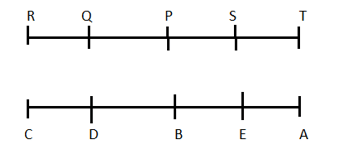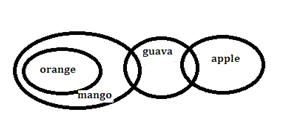# Reasoning Quiz for IBPS CLERK & LIC ASS. PRE 2019 | 24 October 2019

## Reasoning Quiz for IBPS CLERK & LIC ASS. PRE

Reasoning Quiz to improve your  Reasoning  for SBI Po & SBI clerk exam Reasoning, IBPS PO Reasoning , IBPS Clerk Reasoning , IBPS RRB Reasoning, LIC AAO , LIC Assistant,  and other competitive exams.

Directions (Q1-Q5): Study the following information to answer the given questions:

Ten people are sitting in two parallel rows containing five people each, in such a way that there is an equal distance between adjacent persons. In row 1, P, Q, R, S and T are seated and all of them are facing south. In row 2, A, B, C, D and E are seated and all of them are facing north. Therefore, in the given seating arrangement, each member seated in a row faces another member of the other row.

D sits third to the left of A. P faces immediate neighbor of D. R sits second to the right of P. Only one person sits between Q and S. B and E are immediate neighbors. E does not face P and Q.

Q1.How many persons are seated between Q and T?

(a) None

(b) One

(c) Two

(d) Three

(e) Cannot be determined

Q (1 – 5):Q1. Ans.(c)

P and S

Q2.Four of the following five are alike in a certain way and thus form a group. Which is the one that does not belong to that group?

(a)R

(b) S

(c)C

(d) T

(e)A

Q (1 – 5):Q2. Ans.(b)

All other are sitting at the ends.

Q3. Who among the following are sitting exactly in the middle of the rows?

(a)P,E

(b) S, D

(c) S, A

(d)A, R

(e) P, B

Q (1 – 5):Q3. Ans.(e)

Q4. Which of the following is true regarding B?

(a) A and C are immediate neighbors of B.

(b) B sits at one of the extreme ends of the line.

(c) Q faces B.

(d) T is an immediate neighbor of the person facing B.

(e) D sits on the immediate left of B.

Q (1 – 5):Q4. Ans.(e)

Q5. Four of the following five are alike in a certain way and thus form a group. Which is the one that does not belong to that group?

(a) T— E

(b) Q — C

(c) S — B

(d)R—A

(e) P — D

Q (1 – 5):Q5. Ans.(d)

In all other, the second is the neighbor of the one facing the first.

Directions (Q6-Q10): Each question consists of some statements followed by some conclusions.Consider the statements to be true even if they are in variance with the commonly known facts. Read all the conclusions and then decide which of the given conclusions logically follow from the given statements and accordingly mark your answer :–

Q6.

Statements :
All sofa are drum.
Some drum are cover.
No cover is a pillow.
Conclusions :
I. All drum can never be pillow.
II. At least some drum are sofa.

A) Either conclusion I or conclusion II follows.

B) Both conclusion I and conclusion II follow.

C) Neither conclusion I nor conclusion II follows.

D)  Only conclusion I follow.

E) Only conclusion II follows.

Q6. Ans(B)Q7.

Statement:
All orange are mango.
Some mango are guava.
Some guava are apple.
Conclusions:
I. Some apple are mango.
II. Some apple are orange.
III. Some guava are mango.

A) None follows.

B) Only I follow.

C) Only II follows.

D) Only III follow.

E) Only II and III follows.

Q7. Ans(D)Q7. Ans(D)Q8.

Statements:
All Charu are Annu.
Some Sneha are Charu.
Some Seena are Annu.
Conclusions:
I. No Seena is a Sneha is a possibility.
II. Some Charu which are Annu they must be a part of Sneha.

A) Either conclusion I or conclusion II follows.

B) Neither conclusion I nor conclusion II follows.

C) Both conclusion I and II follows.

D)  Only conclusion II follows.

E) Only conclusion I follow.

Q8. Ans(E)Q9.

Statements:
All Om are Shiv.
All Guru are Om.
All Shiv are Saint.
Conclusions:
I. All Saint are Guru is a possibility.
II. Some Saint which are Om also a part of Guru.

A) Either conclusion I or conclusion II follows.

B) Neither conclusion I nor conclusion II follows.

C) Both conclusion I and II follows.

D)  Only conclusion II follows.

E) Only conclusion I follow.

Q9. Ans(E)Q10.

Statements:
All Smith are Lee.
All Amla are Ricky.
No Amla is Lee.
Conclusions:
I. All Smith are Ricky is a possibility.
II. All Lee which are Ricky are also a part of Amla.

A) Either conclusion I or conclusion II follows.

B) Neither conclusion I nor conclusion II follows.

C) Both conclusion I and II follows.

D)  Only conclusion II follows.

E) Only conclusion I follow.

Q10. Ans(E)### You Can Read This Also:

Best E-books for LIC AAO 2019 : Get PDF here

ambitiousbaba.com need your support to Grow

I challenge you will get Best Content in Our PDFs with Detail solutions and Latest Pattern

Memory Based Puzzle E-book | 2016-19 Exams Covered

Get PDF here

Caselet Data Interpretation 200 Questions

Get PDF here
Puzzle & Seating Arrangement E-Book for BANK PO MAINS (Vol-1)

Get PDF here

### ARITHMETIC DATA INTERPRETATION 2019 E-book

Get PDF here
The Banking Awareness 500 MCQs E-book| Bilingual (Hindi + English)

Get PDF here

High Level DATA INTERPRETATION Practice E-BOOK

Get PDF her

3